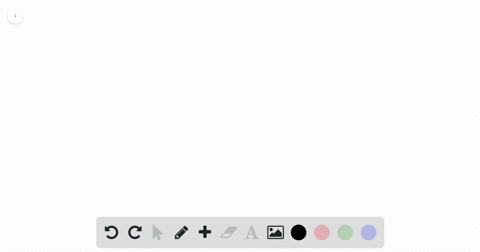Enroll in one of our FREE online STEM summer camps. Space is limited so join now!View Summer Courses### Combat Model. A simplified mathematical model for…

01:21Georgia Southern University

Need more help? Fill out this quick form to get professional live tutoring.

Get live tutoring
Problem 17

Using the vectorized Runge-Kutta algorithm, approximate the solution to the initial value problem
$$\begin{array}{ll}{\frac{d u}{d x}=3 u-4 v ;} & {u(0)=1} \\ {\frac{d v}{d x}=2 u-3 v ;} & {v(0)=1}\end{array}$$
at $x=1$. Starting with $h=1$ continue halving the step size until two successive approximations of $u(1)$ and $v(1)$ differ by at most 0.001.

0.2719

## Discussion

You must be signed in to discuss.

## Video Transcript

No transcript available

#### You're viewing a similar answer. To request the exact answer, fill out the form below:

Our educator team will work on creating an answer for you in the next 6 hours.